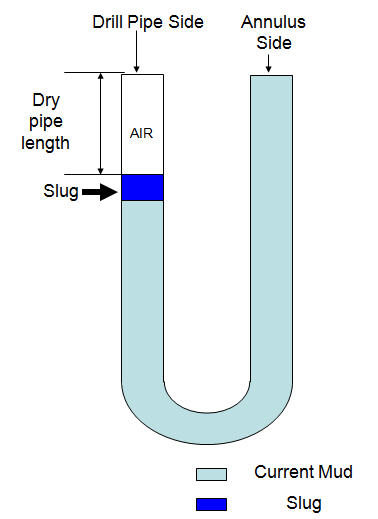# Weight of slug required for desired length of dry pipe with set volume of slug

You can determine how much slug weight required in order to achieve desired length of dry pipe with certain slug volume that you will use.## Oilfield Unit

Step 1 Determine Length of slug in drill pipe in ft:

Length of slug in drill pipe in ft = slug volume in bbl ÷ drill pipe capacity in bbl/ft

Step 2 Determine hydrostatic pressure required to give desired dry pipe drill pipe:

Hydrostatic Pressure in psi = mud weight in ppg × 0.052 × desired length of dry pipe

Step 3 Determine slug weight needed in ppg:

Slug weight in ppg = (Hydrostatic Prำssure (from step 2) ÷ 0.052 ÷ Length of slug in ft (step1)) + mud weight, ppg, in hole

Example: Determine slug weight required for the following data:

Desired length of dry pipe = 200 ft

Mud weight in hole = 12.0 ppg

Drill pipe capacity = 0.016 bbl/ft

Volume of slug = 20 bbl

Step 1 – Determine Length of slug inside drill pipe in ft:

Slug length = 20 bbl ÷ 0.016 bbl/ft

Slug length = 1250 ft

Step 2 – Determine hydrostatic pressure required to give desired dry pipe drill pipe

Hydrostatic Pressure in psi = 12.0 × 0.052 × 200

Hydrostatic Pressure in psi = 124.8 psi

Step 3 – Determine slug weight needed in ppg:

Slug weight in ppg = (124.8 ÷ 0.052 ÷ 1250) + 12.0

Slug weight in ppg = 13.9 ppg

## Metric Unit

Step 1 Determine Length of slug in drill pipe in meter (m):

Length of slug in drill pipe in m = slug volume in m³ ÷ drill pipe capacity in m³/m

Step 2 Determine hydrostatic pressure required to give desired dry pipe drill pipe:

Hydrostatic Pressure in psi = mud weight in kg/m³ × 0.00981 × desired length of dry pipe in m

Step 3 Determine slug weight needed in ppg:

Slug weight in ppg = (Hydrostatic Pressure (from step 2) ÷ 0.00981÷ Length of slug in m (step1)) + mud weight, kg/m³, in hole

Example: Determine slug weight required for the following data:

Desired length of dry pipe = 120 m

Mud weight in hole = 1,400 kg/m³

Drill pipe capacity = 0.007824 m³/m

Volume of slug = 4.5 m³

Step 1 – Determine Length of slug inside drill pipe in ft:

Slug length = 4.5 m³÷ 0.007824 m³/m

Slug length = 575 m

Step 2 – Determine hydrostatic pressure required to give desired dry pipe drill pipe

Hydrostatic Pressure in psi = 1,400 × 0.00981 × 120

Hydrostatic Pressure in psi = 1,648 KPa

Step 3 – Determine slug weight needed in ppg:

Slug weight in ppg = (1,648 ÷ 0.00981 ÷ 575) + 1,400

Slug weight in ppg = 1692 kg/m³Please find the excel sheet used to calculate Weight of slug required for a desired length of dry pipe with a set volume of slug.Ref books:

Lapeyrouse, N.J., 2002. Formulas and calculations for drilling, production and workover, Boston: Gulf Professional publishing.

Bourgoyne, A.J.T., Chenevert , M.E. & Millheim, K.K., 1986. SPE Textbook Series, Volume 2: Applied Drilling Engineering, Society of Petroleum Engineers.

Mitchell, R.F., Miska, S. & Aadny, B.S., 2011. Fundamentals of drilling engineering, Richardson, TX: Society of Petroleum Engineers.

Share the joy1.Arif says: